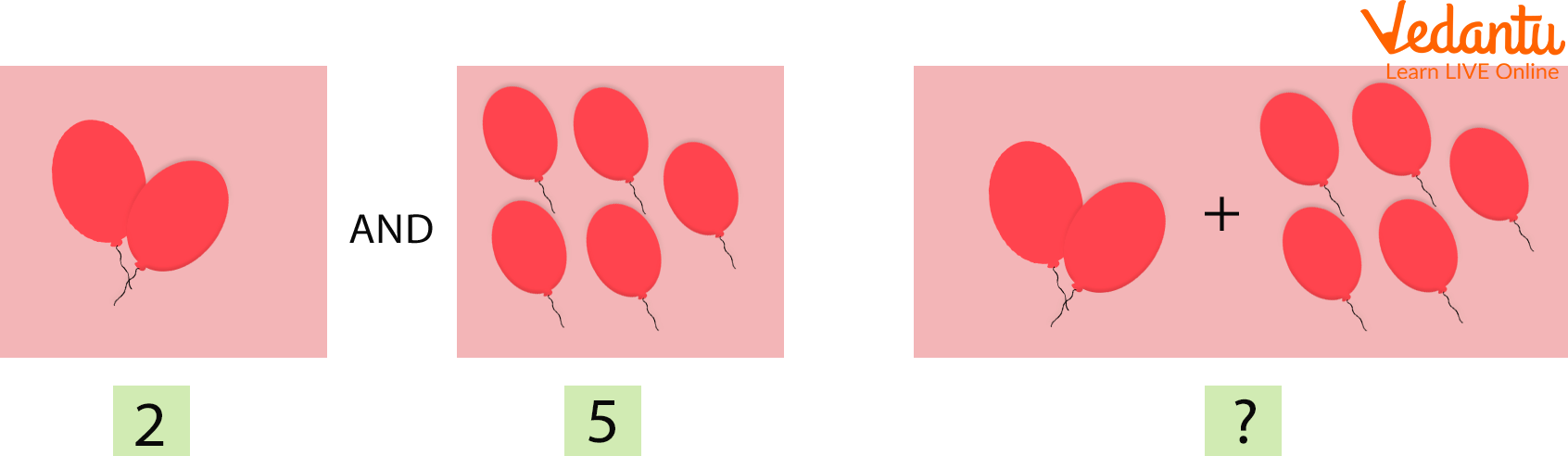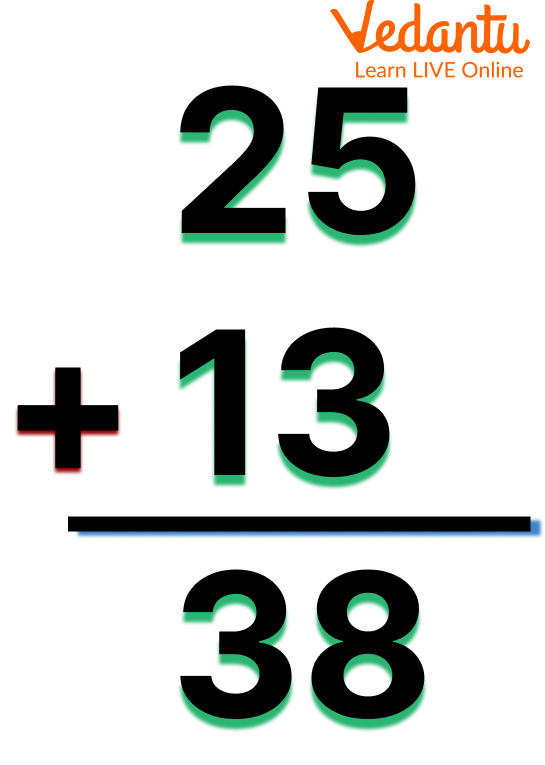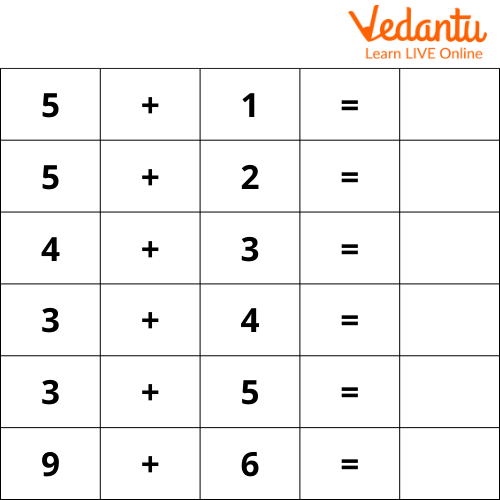Courses
Courses for Kids
Free study material
Free LIVE classes
More## Learning and Doing Addition for Class 1

Addition happens when you put two or more numbers together to find the total amount. The result of adding two or more numbers is called the sum. So, you would add your 4 cookies and your friend's 4 cookies to find that the sum is 8 cookies.

Addition is a mathematical operation that is used to add numbers. The sum of the provided numbers is the outcome of adding the given numbers. For instance, if we add 2 and 3, (2 + 3), the result is 5. We used the addition function on two numbers, 2 and 3, to get the sum, 5. In this article, we are going to see problems based on addition like the word problem of addition for class 1.

Different symbols are used in Mathematics. One of the most used arithmetic symbols is the addition symbol. We read about adding two integers, 2 and 3, in the definition of addition above. If we look at the addition pattern (2 + 3 = 5), we can see that the symbol (+) joins the two numbers and completes the supplied phrase. The addition symbol is made up of one horizontal and one vertical line. It is also known as the plus symbol (+) or the addition sign (+).

## Simple Addition for Class 1

The basic formula of easy addition for Class 1 or the mathematical equation of addition can be explained as follows. Here, 2 and 5 are the addends and 7 is the sum.## 2 Digit Addition for Class 1

2-digit addition for Class 1 is a simple form of addiction in which numbers are placed according to their place value of ones and tens and then added. Once both columns are added, we obtain the final sum. For finding a total of two single-digit numbers, just count the first number on your fingers and then count the second number on your finger, and the sum of the total fingers is the result.## Carryover Addition for Class 1

When adding numbers, if the sum of the addends in any of the columns is more than 9, we regroup this amount into tens and ones. The tens digit of the sum is then carried over to the preceding column, and the one's digit of the sum is written in that column. In other words, we just write the number in the 'ones place digit' in that column, while moving the number in the 'tens place digit' to the column to the immediate left. With the help of an example, let us learn how to add two or more numbers in carrying over addition for Class 1.

Solution: Let us follow the given steps:

Step 1: Start with the digits in one place. (5 + 5 = 10). Here the sum is 10. The tens digit of the sum, that is, 1, will be carried to the preceding column.

Step 2: Add the digits in the tens column along with the carryover 1. This means, 1 (carry-over) + 7 + 6 = 14. Here the sum is 14. The tens digit of the sum, that is, 1, will be carried to the hundreds place.

Step 3: Now, add the digits in the hundreds place along with the carryover digit 1. This means, 1 (carry-over) + 4 + 8 = 13. Here the sum is 13. The tens digit of the sum, that is, 1, will be carried to the thousands place.

Step 4: Now, add the digits in the thousands place along with the carryover digit 1, that is, 1 (carry-over) + 3 + 2 = 6

Step 5: Therefore, the sum of 3475 + 2865 = 6340

## Addition Story Sums for Class 1

One morning, your mother called you and gave you 4 chocolates. After that, your father called you and gave you 5 chocolates. Amazing isn’t it? Now you have so many chocolates. Can you tell me how many chocolates you have? If you have to answer this question then you need to use addition.

Addition is basically counting the total number of objects you have. While adding two numbers, count forward the numbers equal to the second number after the first number. Addition will be easy if we take the bigger number and then count forward the smaller number. While adding 5 + 4 counting four numbers after 5 is easier than counting five numbers after 4. So the answer is 9 chocolates.

Learning becomes easy if you imagine stories in your mind and then think accordingly. Solving the word problems on addition for Class 1 will help you to understand addition in the real world.

Example 1: Peter has two boxes of Chocolates. There are 25 chocolates in one box and 15 chocolates in the other box. How many Chocolates does Peter have in total?

Solution: 25 Chocolates in one box and 15 chocolates in the other box makes a total of 40 chocolates in both boxes.

Example 2: Sam went to a park, 8 boys and 10 girls were already playing there when Sam came to the park. How many children were playing in the Park when Sam came to the park?

Solution: On adding 8 boys and 10 girls together, we get 18. So, when Sam came to the park, he saw 18 Children Playing.

## Solved Examples

Ans: Adding both we get 250.

Ans: Adding both we get 57.

Ans: Adding both we get 75.

Q4. Add 73 + 76 using the carry method.

Ans: Adding both we get 149

Q5. Add 59 + 94 using the carry method.

Ans: Adding both we get 153

## Practice Questions

Some practice questions for simple addition Class 1.Practice Question

## Summary

The process of combining two or more items is known as an addition. The procedure of determining the sum of two or more numbers in Mathematics is known as addition. It is a basic mathematical operation that we all employ on a daily basis. Addition (usually denoted by a plus sign +) is one of the four basic operations of arithmetic, the other three being subtraction, multiplication, and division. Also, we have seen some word problems related to the addition of numbers. We hope this article will help you in understanding the addition for Class 1.

Last updated date: 02nd Oct 2023
Total views: 113.4k
Views today: 3.13k

## FAQs on Addition for Class 1

1. What are the real-life examples of addition?

There are plenty of other examples that we face on a daily basis. If you have 5 apples and a buddy gives you 3 more, the sum of 5 + 3 equals 8. So you have a total of eight apples. Similarly, imagine a class that has 16 females and 13 boys; adding the numbers 16 + 13 yields the total number of students in the class, which is 29.

2. Where do we use addition?

In everyday settings, we apply addition. For example, if we want to know how much money we spent on the products we purchased, the time it would take to complete a task or the number of materials needed in preparing anything, we must do the additional process.

3. What are some of the tips and tricks for an addition?

The following are some of the additional tips and tricks:

• Words like 'put together, 'in all, 'altogether', and 'total' give a clue that you need to add the given numbers.